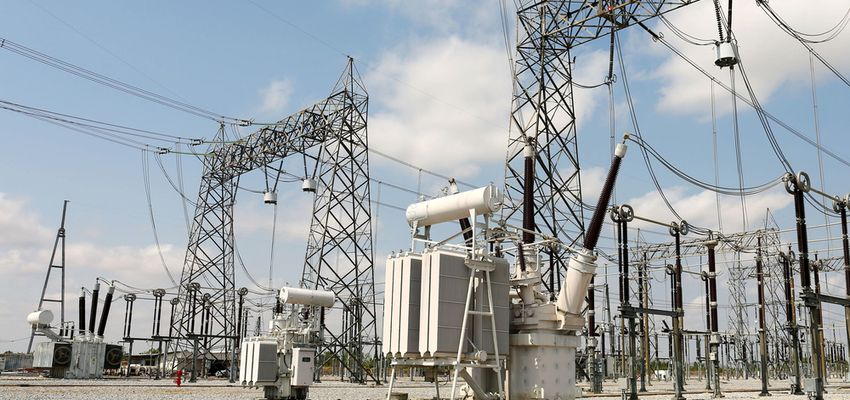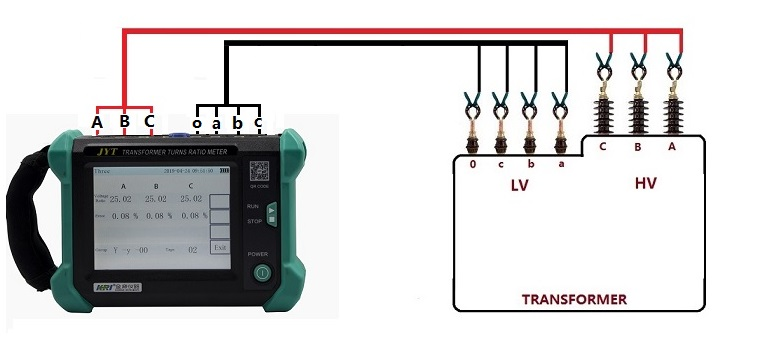Technology Literature

# How to Choose the Right Turns Ratio of CT/PT?

## How to choose turns ratio of current transformer(CT)?

The rated current value of the secondary side of the current transformer(CT) is 5A or 1A, and 5A is generally selected. The commonly used turns ratios for measuring current transformer(CT)s are 5/5, 10/5, 15/5, 20/5, 25/5, 30/5, 40/5, 50/5, 75/5, 100/5, 150 /5, 200/5, 250/5, 300/5, 400/5, 500/5, 600/5, 750/5, 800/5, etc., then how to correctly choose the transformation turns ratio of the current transformer(CT)?

According to the provisions of IEC60044, under the operating conditions of the rated value, the indication of the tester should be at 70%~100% of the tester range. At this time, the maximum transformation turns ratio of the current transformer(CT) should be:

N=I1RT /(0.7*5);

I1RT ---- rated current of primary side of transformer, A;

N----transformation turns ratio of current transformer(CT);

That is to say, it can generally be selected according to the actual current being 80% of the primary current of the transformer.

The selection turns ratio of the current transformer(CT) should also be combined with the accuracy, the actual load and the requirements of the protection device

The Voltage Transformer(PT) with a transformation turns ratio of 10000/100 and a current transformer(CT) with a transformation turns ratio of 100/5 have an extended range. The measured reading of the voltmeter is 10v and the reading of the ammeter is 5A. What are the voltage and current of the circuit under test?

Answer:10000/100 is 100 times, the measured secondary voltage is 10V, then the primary voltage is 1000V.

100/5 is 20 times, the measured secondary current is 5A, then the primary current is 100AIn short, the rated current of the current transformer(CT) cannot be less than your actual measurement current (that is, where you want to install the transformer, you must know the current there), it must not be less than the test current, but it cannot be too large than the actual current. More, generally controlled at 1.5 of the actual current to accompany.

There is also a simple way to see if there is an air switch on the upper or lower end of the transformer you want to install. If there is, see how much A of the air switch is. For example: if the air switch is 300A, then choose the transformation turns ratio as 300/ 5 transformers.

#### What happens if the transformation turns ratioof the current transformer(CT)is selected to be smaller?

This does not have a big impact. Although the rated current exceeds the primary rated current of the CT in terms of rated data, from the perspective of actual opeturns ration, the transformer will generally not run at full load, sometimes reaching 60~70% of the rated power. %, so the current transformer(CT) generally does not work at full load or overload. However, if the transformer has been working at full load, it will affect the measurement accuracy of the CT and the watt-hour meter.

### Does the larger current transformer(CT)turns ratiohave any effect on the measurement?

It depends on how big it is.

First, the general transformer can guarantee the linearity with excellent accuracy within the range of 12%~90% of its rated current. Therefore, most of the time when choosing a transformer, it will be appropriately larger.

Second, the turns ratio tester also has good accuracy within a certain range. The general choice is: it is ideal to keep the working current at about 30% to 75% of its rated current.

If the transformer turns ratio is too large, the current flowing through the tester will be too small, and the test speed will be slowed down.

Therefore, if the current transformer(CT) turns ratio is too large or too small, it will have an adverse effect on the measurement.

## Selection of Transformer turns ratioof Voltage Transformer(PT)

The transformation turns ratio of the Voltage Transformer(PT) is the turns ratio of the primary voltage and the secondary voltage. When the primary voltage is applied, the secondary voltage is converted into a low voltage according to the transformation turns ratio, which is convenient for measurement and electric energy measurement.

In the neutral point direct grounding system of 110kV and above voltage level, the commonly used Voltage Transformer(PT) has two secondary windings: the main secondary winding and the auxiliary (open delta) secondary winding, as shown in Figure 1. The rated phase voltage of the main secondary winding is 100/31/2V, and the rated phase voltage of the auxiliary (open delta) secondary winding is 100V. Voltage Transformer(PT) transformation turns ratio Ku (Un/31/2)/(100/31/2) (Un is the rated voltage of the primary system).

In the neutral point indirect grounding system with voltage level of 35kV and below, the commonly used Voltage Transformer(PT) also has two secondary windings, of which the rated phase voltage of the main secondary winding is 100/31/2V, and the auxiliary (open delta) two The rated phase voltage of the secondary winding is 100/3V. The Voltage Transformer(PT) transformation turns ratio Ku is (Un/31/2)/(100/31/2)/(100/3).

## JYT Handheld transformer turn ratio tester: